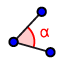# Triangle Centers worksheet

Topic:
Geometry

## Question 1

In the GeoGebra applet above, the points D, E, and F represent the centroid, circumcenter, and orthocenter of the triangle ABC. (Not necessarily in that order.) Determine which point is which.

Select all that apply
• A
• B
• C
• D
• E
• F

## Question 2

These three centers (Centroid, Circumcenter, Orthocenter) share a special property. In the applet above, move the points A, B, and C to see what changes, and what stays the same over lots of different triangles. Make a conjecture about the points D, E, and F.

• Hint: Trying holding a ruler up to the screen.

## Task #2: Location for a new shopping center

You are planning to build a shopping center between towns A, B, and C. These towns are very competitive, and if the shopping center is closer to one town than another, the farther-away town won't shop there. (For example, if the shopping center is 5 miles from towns A and B, and 8 miles from town C, town C won't shop there.) Construct a location for your shopping center that will make towns A, B, and C happy.

## Question 3

What is the geometry name for the location you chose for the shopping center? Why did you make that choice?

## Task #3: Location for a Water Treatment Center

You are planning to build a Water Treatment Center that will serve towns A, B, and C. In this task, your goal is NOT to make sure the treatment center is equidistant from A, B, and C, but to minimize the total distance from the treatment center, D, to each of the three towns. Complete the tasks listed below this applet.

Move the point D to a position that minimizes Total Distance. Drag the point D around until you get "Total distance" as small as possible. Hint: The minimum total distance is very close to 17 units.

To explore the position that made this minimization possible, measure the angles ADB, BDC, and CDA.To find the angles, click the "Angle" tool, then select, IN ORDER, the points A, D, B. Then, select B, D, C. Then, select C, D, A.

## Question 4

The point that minimizes total distance to the vertices of a triangle is called the triangle's Fermat point. Based on your observations, make a conjecture about the Fermat point of a triangle.

## Task #4: Find the Fermat point

Use the instructions below to construct the Fermat point of the triangle ABC.

## Instructions:

1.Select the "Regular Polygon" tool, then select the points A, B (in that order). When prompted, enter "3" vertices. You have made an equilateral triangle on side AB. (Let's call it ABD.)
2.Repeat the last steps to make an equilateral triangle on side BC. (Let's call it BCE.)
3.Repeat again to make a third equilateral triangle on side CA. (Let's call it CAF.)
4.Construct lines from the third (non-ABC) vertex of each equilateral triangle to the opposite vertex on AB. For example, the first line should pass through points D and C.
5.Use the intersect tool to construct the intersection of these lines. This is the Fermat point!